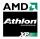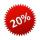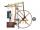Bureaucracy

The office was attended by 23 people. 9 of them completed the questionnaire.

What is the percentage of all people?

Result

p =  39.1 %

Solution:Leave us a comment of this math problem and its solution (i.e. if it is still somewhat unclear...):Be the first to comment!To solve this verbal math problem are needed these knowledge from mathematics:

Our percentage calculator will help you quickly calculate various typical tasks with percentages.

Next similar examples:

1. Sales offGoods is worth € 70 and the price of goods fell two weeks in a row by 10%. How many % decreased overall?
2. ClassIn 7.C clss are 10 girls and 20 boys. Yesterday was missing 20% of girls and 50% boys. What percentage of students missing?
3. ClassIn a class are 32 pupils. Of these are 8 boys. What percentage of girls are in the class?
4. Human buying irrationalityI longer watch processors for Socket A on ebay, Athlon XP 1.86GHz with a PR rating of 2500+ costs \$7 and Athlon XP 2.16Ghz with a PR rating of 3000+ currently cost \$16. Calculate: About what percentage of the Athlon XP 2.16Ghz is powerful than Athlon X
5. NumberWhat number is 20 % smaller than the number 198?
6. RatioIncrease in the ratio 20:4 number 18.5.
7. Ratio v2Decrease in the ratio 12:16 number 13.2.
8. Seeds 2How many seeds germinated from 1000 pcs, when 23% no emergence?
9. IronIron ore contains 57% iron. How much ore is needed to produce 20 tons of iron?
10. Percentages and numbersHow many percent is number 426 greater than number 71?
11. EfficiencyA certain device consumes 28 kW and delivers 14 kW. Find its efficiency.
12. Arble bagA marble bag sold by Rachel's Marble Company contains 5 orange marbles for every 6 green marbles. If a bag has 35 orange marbles, how many green marbles does it contain?
13. Energy savingThey were released three different, independent inventions saving 12%, 15% and 25% energy. Some considered that while the use of these inventions, the total savings will be 12% + 15% + 25% = 52%. Is this true? How much percent of energy will save all thre
14. Base, percents, valueBase is 344084 which is 100 %. How many percent is 384177?
15. Apples 2James has 13 apples. He has 30 percent more apples than Sam. How many apples has Sam?
16. Percents - easyHow many percent is 432 out of 434?
17. Percentage 1052 shorts and missed 13. Calculate percentage